# 10.2.2 Transformations II, PT3 Focus Practice

 
Question 6:
Diagram below shows a ‘Wayang Kulit’ performed by Tok Dalang. The height of the screen use is 1.5 m. The shadow on the screen is ⅔ the height of the screen.What is the height, in cm, of the puppet used by Tok Dalang?

Solution:
 

 
 
 
Question 7:
Diagram below shows the shadow of a pillar form on the wall from the light of a spotlight.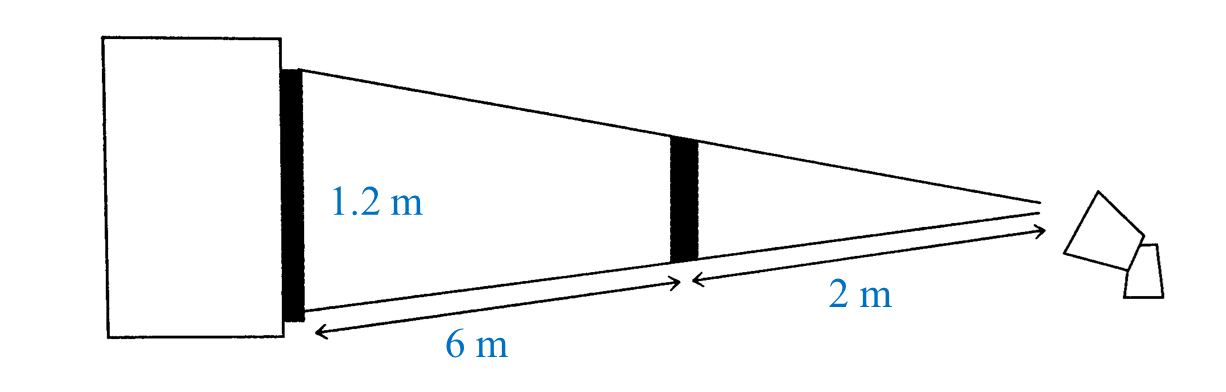(a) State the scale factor of the enlargement.
(b) Find the height of the pillar.

Solution:
(a)

(b)

 
 
 
Question 8:
In diagram below, a torch light is used to form a shadow of a candle on the screen.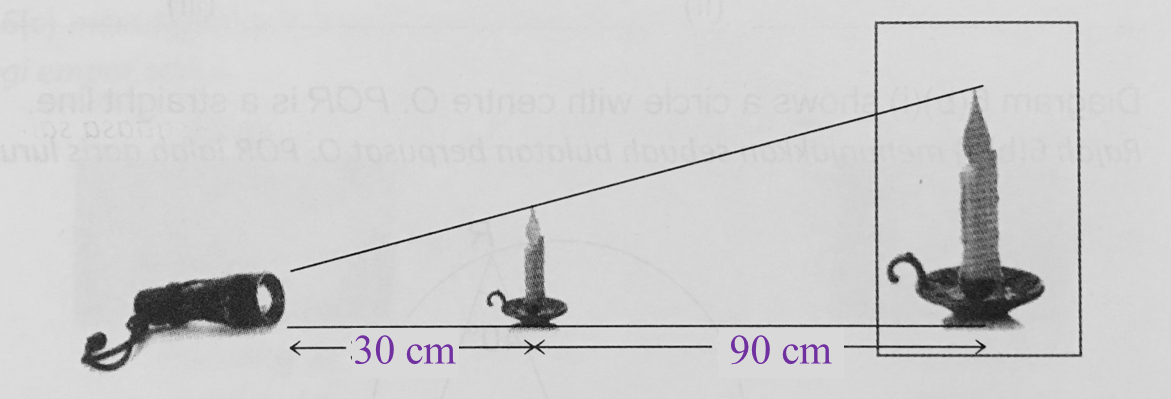The height of the candle is 15 cm.
If the height of the shadow of the candle is ¾ of the height of the screen, find the height of the screen.

Solution:
 

 
 

# 10.2.1 Transformations II, PT3 Focus Practice

     
10.2.1 Transformations II, PT3 Focus Practice
     

   Question 1:
   
In the diagram, ∆P’Q’R’ is the image of ∆PQR under an enlargement.
   
   
       
   

   
   
(a) State the scale factor of the enlargement.
   
(b)   If the area of ∆PQR = 7 cm2, calculate the area of ∆P’Q’R’.
   
   

    Solution:
   
(a)
   
       
   

    (b)
   
Area of image = k× Area of object
   
Area of ∆P’Q’R’ = 22 × 7
   
   
= 28 cm2
 
 
 
 
     
   
   
Question 2:
   
In diagram below, OP’Q’ is the image of OPQ under an enlargement with centre O.
 
 
   
       
Given PQ = 4cm, calculate the length, in cm, of P’Q’.
   
   

    Solution:
     

   

     
 
 
     
   
Question 3:
   
In diagram below, PQ’R’S’ is the image of PQRS under an enlargement.
   

   
       
Calculate the length, in cm, of SS’.
   
   

    Solution:
     

   

   
 
     
   
Question 4:
   
On the Cartesian plane, Q’R’S’ is the image of ∆ QRS under an enlargement of centre T.
   
       

   
State the coordinates of T.
   
   

    Solution:
   
 
         

   
Coordinates of T = (4, 4).
 
 
 
 
     
   
Question 5:
   
In diagram below, quadrilateral AFGH is the image of ABCD under an enlargement.
   
   
   
   
 (a) Find the scale factor of the enlargement.
   
(b) The area represented by the quadrilateral AFGH is 15cm2. Find the area, in cm2, represented by the shaded region.
   
   

    Solution:
   
(a)
   
    $\begin{array}{l}\text{Scale factor}\\ =\frac{3}{6+3}=\frac{3}{9}=\frac{1}{3}\end{array}$    
   
   

    (b)
   
Area of image = k× Area of object
   
       
   

 
   

# 10.1 Transformations II

10.1 Transformations II

10.1.1 Similarity
Two shapes are similar if
(a) the corresponding angles are equal and
(b)   the corresponding sides are proportional.

Example:

$\begin{array}{l}\angle A=\angle J={90}^{o}\\ \angle B=\angle K={50}^{o}\\ \angle C=\angle L={130}^{o}\\ \angle D=\angle M={90}^{o}\end{array}$

(All the corresponding angles are equal.)

$\begin{array}{l}\frac{AB}{JK}=\frac{5}{10}=\frac{1}{2}\\ \frac{BC}{KL}=\frac{4}{8}=\frac{1}{2}\\ \frac{CD}{LM}=\frac{2.5}{5}=\frac{1}{2}\\ \frac{AD}{JM}=\frac{3}{6}=\frac{1}{2}\end{array}$

(All the corresponding sides are proportional.)

10.1.2 Enlargement
1. Enlargement is a type of transformation where all the points of an object move from a fixed point at a constant ratio.

2. The fixed point is known as the centre of enlargement and the constant ratio is known as the scale factor.
$\text{Scale factor}=\frac{\text{length of side of image}}{\text{length of side of object}}$

3.
The object and the image are similar.

4. If A’ is the image of A under an enlargement with centre O and scale factor k, then $\frac{OA\text{'}}{OA}=k$
• if k > 0, then the image is on the same side of the object.
• if k < 0, then the image is on the opposite side of the object.
• if –1 < k < 1, then the size of the image is a reduction of the size of the object.
5. Area of image = k2 × area of object.

# 9.2.2 Scale Drawings, PT3 Focus Practice

Question 6:
Diagram below shows an irregular pentagon.By using the scale 1 : 200, complete the scale drawing in the answer space provided. The grid has equal square with sides of 1 cm.Solution: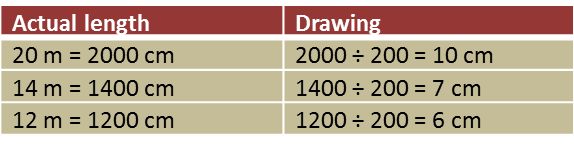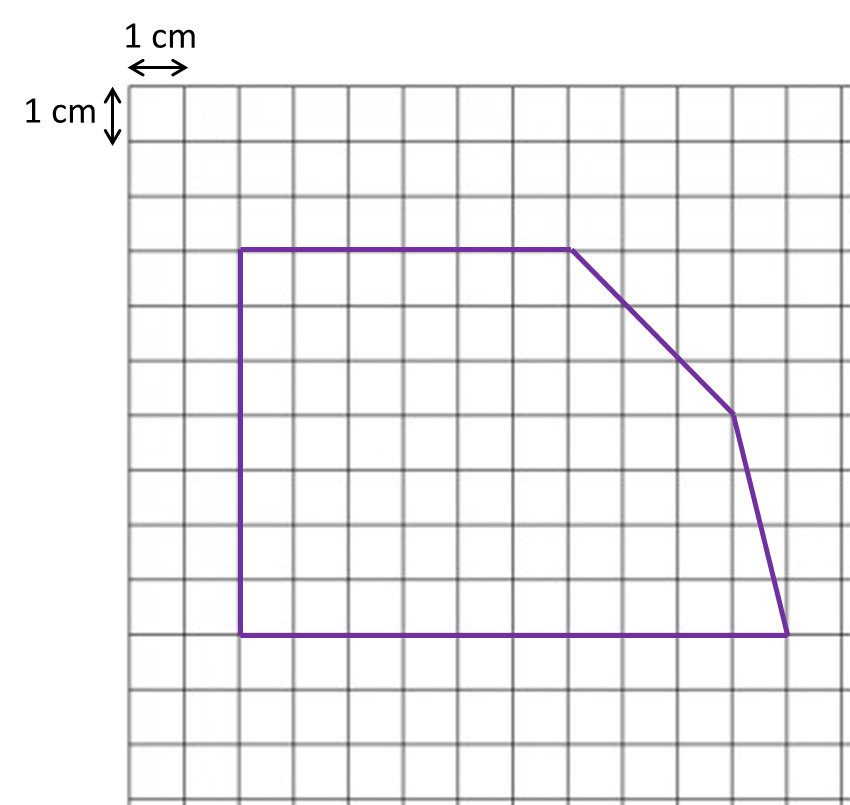Question 7:
Diagram below shows polygon.(a) If the polygon is redrawn using the scale 1 : 500, calculate the length of side drawn for the side 15 m.
(b) On the square grids in the answer space, redraw the polygon using the scale 1 : 500. The grid has equal squares with sides of 1 cm.

(b)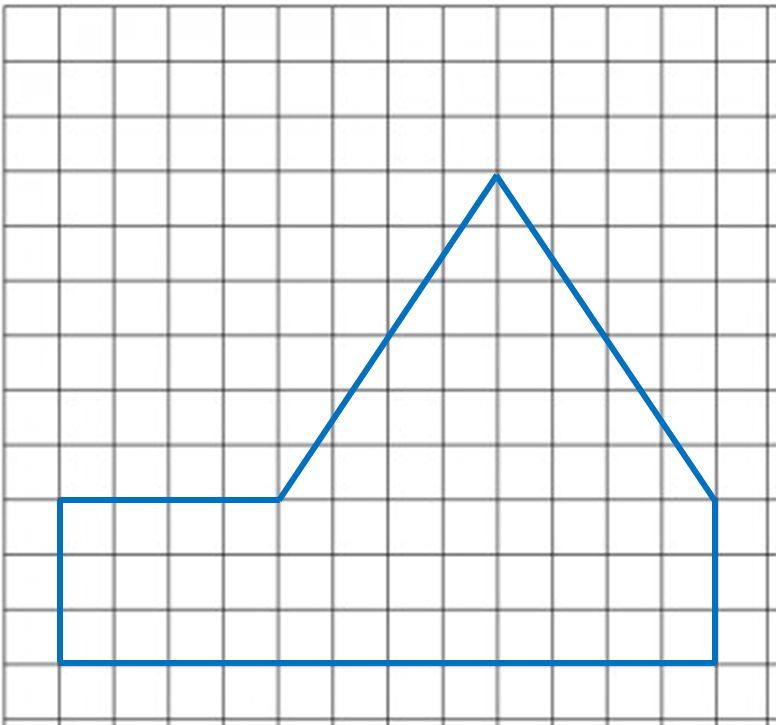# 9.2.1 Scale Drawings, PT3 Focus Practice

Question 1:
Diagram below shows polygon P drawn on a grid of equal squares with sides of 1 unit.On the grid in the answer space, redraw polygon P using the scale
The grid has equal squares with sides of 1 unit.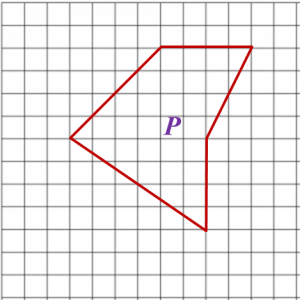Question 2:
Diagram below shows polygon W drawn on a grid of equal squares with sides of 1 unit.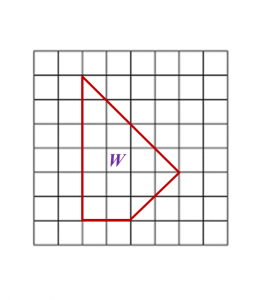On the grid in the answer space, redraw polygon W using the scale 1 : 2.
The grid has equal squares with sides of 1 unit.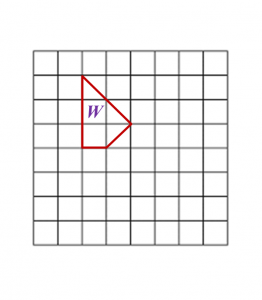Question 3:
Diagram below shows a polygon PQRSTU.(a) If the polygon is redrawn using the scale 1 : 400, calculate the length of PQ.
(b) In the answer space, redraw the polygon using the scale 1 : 400. The grid has equal squares with sides of 1 cm.

(a)

(b)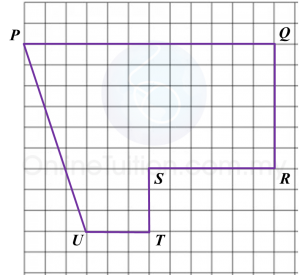Question 4:
Diagram below shows a polygon.On the grid in the answer space, redraw the polygon using the scale 1 : 500. The grid has equal squares with sides of 1 cm.

 Actual length Drawing 45 m = 4500 cm 4500 ÷ 500 = 9 cm 20 m = 2000 cm 2000 ÷ 500 = 4 cm 35 m = 3500 cm 3500 ÷ 500 = 7 cm 10 m = 1000 cm 1000 ÷ 500 = 2 cm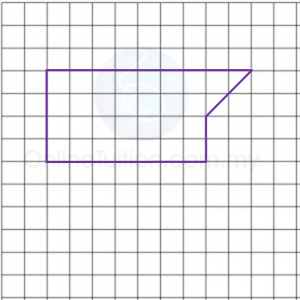Question 5:
Diagram below shows a polygon.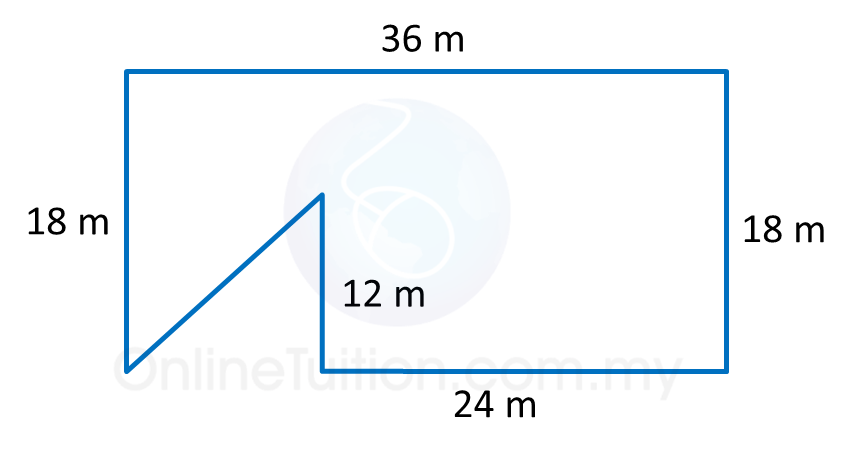(a) If the polygon is redrawn using the scale 1 : 300, calculate the length, in cm, the drawing for the side 24 m.
(b) In the answer space, redraw the polygon using the scale 1 : 300. The grid has equal squares with sides of 1 cm.